## Meters; and RC Circuits

7-11-00

Sections 19.7 - 19.11

It is often useful to measure the voltage or current in a circuit. A voltmeter is a device used to measure voltage, while a meter measuring current is an ammeter. Meters are either analog or digital devices. Analog meters show the output on a scale with a needle, while digital devices produce a digital readout. Analog voltmeters and ammeters are both based on a device called a galvanometer. Because this is a magnetic device, we'll come back to that in the next chapter. Digital voltmeters and ammeters generally rely on measuring the voltage across a known resistor, and converting that voltage to a digital value for display.

### VoltmetersResistors in parallel have the same voltage across them, so if you want to measure the voltage across a circuit element like a resistor, you place the voltmeter in parallel with the resistor. The voltmeter is shown in the circuit diagram as a V in a circle, and it acts as another resistor. To prevent the voltmeter from changing the current in the circuit (and therefore the voltage across the resistor), the voltmeter must have a resistance much larger than the resistor's. With a large voltmeter resistance, hardly any of the current in the circuit makes a detour through the meter.

### Ammeters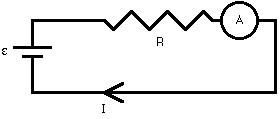Remember that resistors in series have the same current flowing through them. An ammeter, then, must be placed in series with a resistor to measure the current through the resistor. On a circuit diagram, an ammeter is shown as an A in a circle. Again, the ammeter acts as a resistor, so to minimize its impact on the circuit it must have a small resistance relative to the resistance of the resitor whose current is being measured.

### RC Circuits

Resistors are relatively simple circuit elements. When a resistor or a set of resistors is connected to a voltage source, the current is constant. If a capacitor is added to the circuit, the situation changes. In a simple series circuit, with a battery, resistor, and capacitor in series, the current will follow an exponential decay. The time it takes to decay is determined by the resistance (R) and capacitance (C) in the circuit.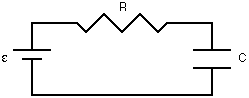A capacitor is a device for storing charge. In some sense, a capacitor acts like a temporary battery. When a capacitor is connected through a resistor to a battery, charge from the battery is stored in the capacitor. This causes a potential difference to build up across the capacitor, which opposes the potential difference of the battery. As this potential difference builds, the current in the circuit decreases.

If the capacitor is connected to a battery with a voltage of Vo, the voltage across the capacitor varies with time according to the equation: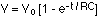The current in the circuit varies with time according to the equation: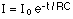Graphs of voltage and current as a function of time while the capacitor charges are shown below.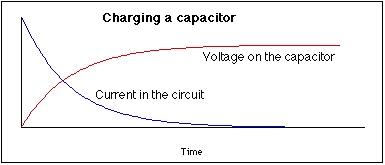The product of the resistance and capacitance, RC, in the circuit is known as the time constant. This is a measure of how fast the capacitor will charge or discharge.

After charging a capacitor with a battery, the battery can be removed and the capacitor can be used to supply current to the circuit. In this case, the current obeys the same equation as above, decaying away exponentially, and the voltage across the capacitor will vary as: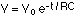Graphs of the voltage and current while the capacitor discharges are shown here. The current is shown negative because it is opposite in direction to the current when the capacitor charges.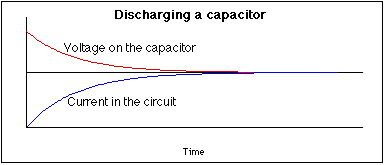### Currents in nerve cells

In the human body, signals are sent back and forth between muscles and the brain, as well as from our sensory receptors (eyes, ears, touch sensors, etc.) to the brain, along nerve cells. These nerve impulses are electrical signals that are transmitted along the body, or axon, of a nerve cell.

The axon is simply a long tube built to carry electrical signals. A potential difference of about 70 mV exists across the cell membrane when the cell is in its resting state; this is due to a small imbalance in the concentration of ions inside and outside the cell. The ions primarily responsible for the propagation of a nerve impulse are potassium (K+) and sodium +.

The potential inside the cell is at -70 mV with respect to the outside. Consider one point on the axon. If the potential inside the axon at that point is raised by a small amount, nothing much happens. If the potential inside is raised to about -55 mV, however, the permeability of the cell membrane changes. This causes sodium ions to enter the cell, raising the potential inside to about +50 mV. At this point the membrane becomes impermeable to sodium again, and potassium ions flow out of the cell, restoring the axon at that point to its rest state.

That brief rise to +50 mV at point A on the axon, however, causes the potential to rise at point B, leading to an ion transfer there, causing the potential there to shoot up to +50 mV, thereby affecting the potential at point C, etc. This is how nerve impulses are transmitted along the nerve cell.

What a nerve cell looks like.
The shape of a nerve impulse.
How a nerve impulse propagates.